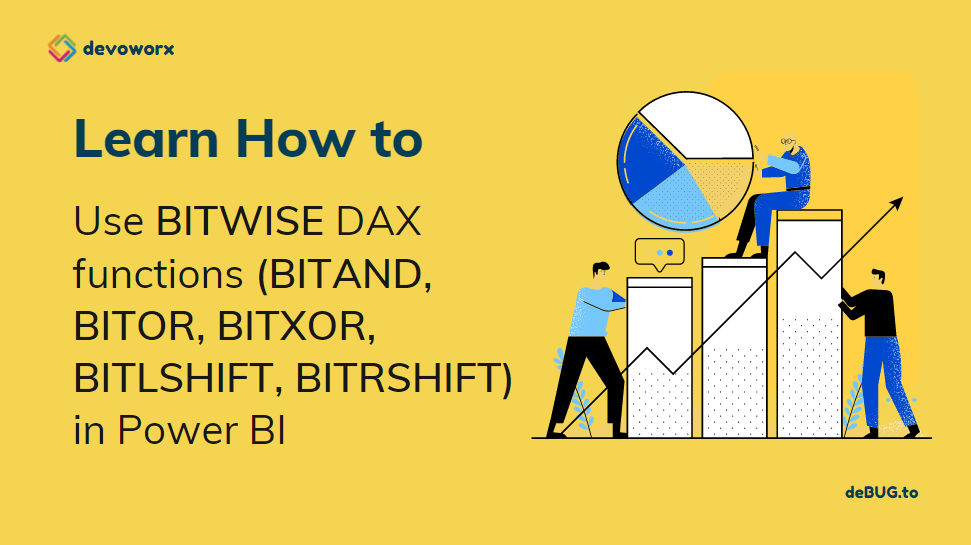Power BI DAX Bitwise Operations

## How to use Power BI DAX Bitwise Operations?

In this post, we will discuss the Power BI DAX bitwise operations “BITAND, BITOR, BITXOR, BITLSHIFT, BITRSHIFT“.

## What is Dax in Power BI?

Data Analysis Expressions (DAX) is a formula expression language explicitly designed for the management of data models, the DAX formulas include functions and operators that can be used to create formulas and expressions in Analysis Services, Power BI, and Power Pivot in Excel.

DAX in Power BI includes a library of over 200 functions, operators, that are used by report designers to perform data analysis and calculations.

## Power BI DAX Bitwise Operations

This section focus on the new Bitwise DAX function that was introduced in November 2021.

The Bitwise DAX function helps you to returns a bitwise of two numbers through using the below additional functions:

1. BITAND.
2. BITOR
3. BITXOR
4. BITLSHIFT
5. BITRSHIFT

## Power BI Bitwise AND (BITAND)

DAX BITAND function is one of the logical functions in DAX that returns a bitwise AND of two numbers.

### Power BI Bitwise AND (BITAND) Syntax

``BITAND(<number1>, <number2>)``

Note: If the value is not an integer, it is truncated.

### Power BI Bitwise AND (BITAND) Examples

Example1:

In this example, you will notice that the BITAND DAX function takes two numbers as integer and convert each number to a binary number then perform AND logical operation then results in a new binary number (1001) that will be converted to an integer value (1001)=9.

``BITAND(11,13) the output will be 9``

Example2:

In this example, consider you have a table with two columns (Number1, and Number2), and each column holds an integer value as shown below:

So in this case, you will be forced to use the BITWISE keyword to can get the column value, and then use the BITAND DAX function as below:

``BITAND(BITWISE[Number1],BITWISE[Number2])``

## Power BI Bitwise OR (BITOR)

DAX BITOR function returns a bitwise OR of two numbers.

Note: If the value is not an integer, it is truncated.

### Power BI BITOR Syntax

``BITOR(<number1>, <number2>)``

Example1

Again, as we earlier explained, the BITOR DAX function takes two numbers as integer and converts each number to binary number then perform OR logical operation then results in a new binary number (1111) that will be converted to an integer value (1111)=15.

``BITOR(13, 11) the output will be 15``

Example2

In this example, consider you have a table with two columns (Number1, and Number2), and each column holds an integer value as shown below:

In this case, you will be forced to use the BITWISE keyword to can get the column value, and then use the BITOR DAX function as below:

``BITOR = BITOR(BITWISE[Number1],BITWISE[Number2])``

## Power BI Bitwise XOR (BITXOR)

DAX BITXOR function returns a bitwise XOR of two numbers.

Note: If the value is not an integer, the function will not work and truncate.

### Power BI BITXOR Syntax

``BITXOR(<number1>, <number2>)``

Example1

the BITXOR DAX function takes two numbers as integer and converts each number to binary number then perform XOR logical operation then results in a new binary number (0110) that will be converted to an integer value (0110)=6.

``BITXOR(13, 11) the output will be 6``

Example2

``BITXOR= BITXOR(BITWISE[Number1],BITWISE[Number2])``

Output

## Power BI Bitwise LSHIFT(BITLSHIFT)

DAX BITLSHIFT function return a number shifted left by the specified number of bits.

### Power BI BITLSHIFT Syntax

``BITLSHIFT(<Number>, <Shift_Amount>) ``

If Shift_Amount is negative, it will shift in the opposite direction.

Example1

``BITLSHIFT(4, 2) the output will be 16``

Example2

``BITLSHIFT= BITLSHIFT(BITWISE[Number1],2)``

Example3

``BITLSHIFT(128, -1) the output will be 64``

## Power BI Bitwise RSHIFT(BITRSHIFT)

DAX BITRSHIFT function returns a number shifted right by the specified number of bits.

### Power BI BITRSHIFT Syntax

``BITRSHIFT(<Number>, <Shift_Amount>) ``

If Shift_Amount is negative, it will shift in the opposite direction.

Example1

``BITRSHIFT(11, 13) the output will be 2``

Example2

``BITRSHIFT= BITRSHIFT(BITWISE[Number1],2)``

#### Conclusion

In conclusion, we have learned How to use the Bitwise DAX function in Power BI by exploring the below related DAX functions

• BITAND.
• BITOR
• BITXOR
• BITLSHIFT
• BITRSHIFT